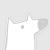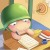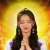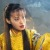Asian Conference on Computer Vision（ACCV2020）将于2020年11月30日至12月4日在京都举行。ACCV是一个两年一度的国际会议，主要由亚洲计算机视觉联合会主办。这一系列会议为研究人员、开发人员和实践者提供了一个重要的论坛，以展示和讨论计算机视觉和相关领域中的新问题、解决方案和技术。官网链接：http://accv2020.kyoto/0+0+0+0+0+0+0+0+0+0+0+0+0+0+0+0+0+0+0+0+0+0+0+0+0+0+0+0+0+0+0+0+0+0+0+0+0+1+0+0+0+0+0+0+0+
Top